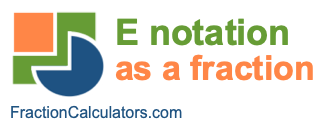E Notation as a Fraction CalculatorWelcome to the E Notation as a Fraction Calculator. This calculator has three functions: It can convert your E notation to a fraction, it can convert your E notation to a decimal number, and it will show you how to write the decimal number you converted from E notation using words.

Please enter the E notation that you want converted to a fraction:

e-

Not sure what the E Notation as a Fraction Calculator can do for you? Check out these examples: